Courses

# Test: Biomolecules- 1

## 35 Questions MCQ Test Organic Chemistry | Test: Biomolecules- 1

Description
This mock test of Test: Biomolecules- 1 for Chemistry helps you for every Chemistry entrance exam. This contains 35 Multiple Choice Questions for Chemistry Test: Biomolecules- 1 (mcq) to study with solutions a complete question bank. The solved questions answers in this Test: Biomolecules- 1 quiz give you a good mix of easy questions and tough questions. Chemistry students definitely take this Test: Biomolecules- 1 exercise for a better result in the exam. You can find other Test: Biomolecules- 1 extra questions, long questions & short questions for Chemistry on EduRev as well by searching above.
QUESTION: 1

Solution:
QUESTION: 2

Solution:
QUESTION: 3

### The most basic amino acid among the following is:

Solution:
QUESTION: 4

Tollens test will be negative for:

Solution:
QUESTION: 5

Specific rotations of freshly prepared aqueous solutions of I and II are +112 and +18.7, respectively. On standing, the optical rotation of aqueous solution of I slowly decreases to give a final value of +52.7 due to equilibrium with II. Under this state of equilibrium, what is the ratio II:I?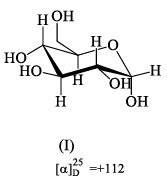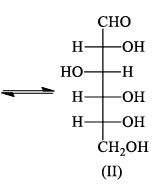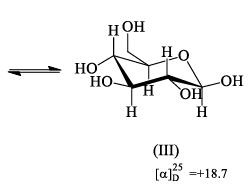Solution:
QUESTION: 6

The Haworth projection for a-anomer of D-glucose is:

Solution:
QUESTION: 7

The correct epimeric pair of the following is: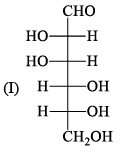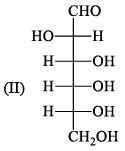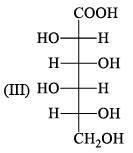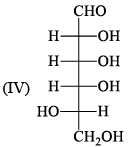Solution:
QUESTION: 8

The incorrect statement in the following is:

Solution:
QUESTION: 9

The titration curve of alanine hydrochloride is given below: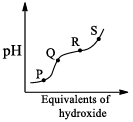The position in the graph that corresponds to the isoelectric point of alanine is:

Solution:
QUESTION: 10

The absolute configurat ions at the two chiral centers in D-ribulose are: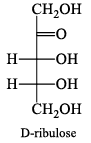Solution:
QUESTION: 11

Among the following compounds, which one on reduction wit h NaBH4 gives meso-compound:

Solution:
QUESTION: 12

The major product of the reaction is: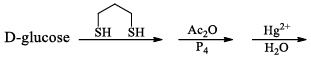Solution:
QUESTION: 13

The final product of the following reaction: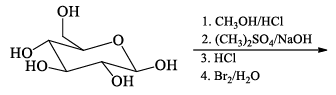Solution:
QUESTION: 14

How many moles of formaldehyde will be formed: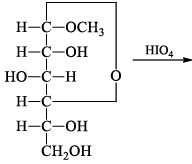Solution:
QUESTION: 15

D-glucose and D-fructose can be distinguished by:

Solution:
QUESTION: 16

Among α- and β-anomeric pairs which one is more stable: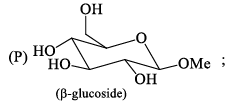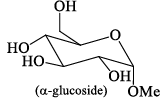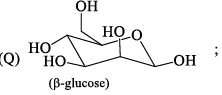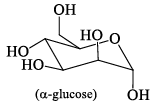Solution:
QUESTION: 17

Among the D-glucopyranose forms given below, which is more stable:

Solution:
QUESTION: 18

Glycosidic linkage will be formed from among which of these reactions:

Solution:
QUESTION: 19

The product of the below reaction would be: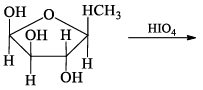Solution:
QUESTION: 20

The compound would be obtained from the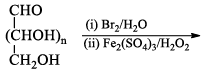Solution:
QUESTION: 21

The below reaction a compound. From the sugars given below, identify the sugar that gives the same compound: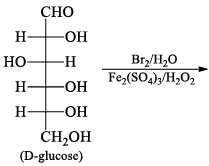Solution:
QUESTION: 22

From the below list, identify the sugars which on reduction give the same glucitol:

Solution:
QUESTION: 23

Generally β-[D] glucose in more stable under H2O. But the solvent is changed to Cyclohexanol under Cyclohexanol. The stable form (major contribution) would be:

Solution:
QUESTION: 24

The structure of D-galactose is: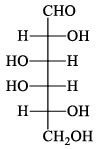Which one of these structures is L-galactose?

Solution:
QUESTION: 25

The complementary strand of DNA for the following single stranded DNA sequence: 5' - A - T - C- A - T - G - C-3' is:

Solution:

Explanation : In DNA, Adenine (A) pairs with Thymine (T) and Guanine (G) pairs with Cytosine (C).

Hence, on replication of DNA in M phase, complementary nucleotides for each base pair will be added as follows:

A - T

T - A

G - C

C - G

Since replication occurs in 5'-3' direction, so the complementary strand will be formed in 3'-5' direction.

QUESTION: 26

Synthesis of each molecule of glucose in photosynthesis involves:

Solution:
QUESTION: 27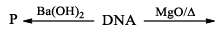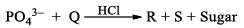In the scheme shown above P, Q, R and S are:

Solution:
QUESTION: 28

The correct statement about the following disaccharide is: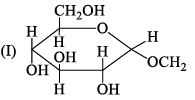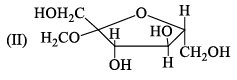Solution:
QUESTION: 29

Match the isoelectric point with the amino acids.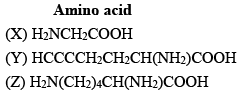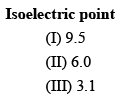Solution:
QUESTION: 30

Cellulose upon acetylation with excess acetic anhydride/H2SO4 (catalyst ic) gives cellulose triacetate whose structure is:

Solution:
QUESTION: 31

The major product of the following reaction is: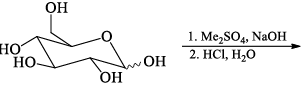Solution:
QUESTION: 32

Amongst the following, the structure of guanosine is:

Solution:
QUESTION: 33

The correct sequence of the amino acids present in the tripeptide given below is: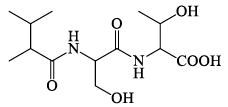Solution:
QUESTION: 34

A disaccharide that will not give Benedict’s test and will not form osazone is:

Solution:
QUESTION: 35

From a carboxymethyl-cellulose column at pH 6.0, Arginine, valine and Glutamic acid will elute in the order:

Solution: